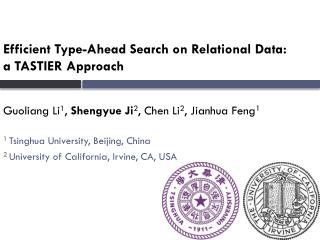Download PresentationEfficient Type-Ahead Search on Relational Data: a TASTIER Approach

# Efficient Type-Ahead Search on Relational Data: a TASTIER Approach - PowerPoint PPT PresentationDownload Presentation## Efficient Type-Ahead Search on Relational Data: a TASTIER Approach

- - - - - - - - - - - - - - - - - - - - - - - - - - - E N D - - - - - - - - - - - - - - - - - - - - - - - - - - -
##### Presentation Transcript

1. Efficient Type-Ahead Search on Relational Data: • a TASTIER Approach • Guoliang Li1, Shengyue Ji2, Chen Li2, Jianhua Feng1 • 1 Tsinghua University, Beijing, China • 2 University of California, Irvine, CA, USA

2. Traditional Keyword Search MUST Type in Complete keywords

3. Type-Ahead Search Advantages: • Interactive: data exploration in relational databases • Full-text search: full-text search on-the-fly

4. Challenges and Preliminaries • Efficiency requirement (milliseconds vs. seconds) • Client-side processing • Network delay • Server-side processing • Opportunities: • Subsequent queries can be answered incrementally

5. Fundamentals • Data • R: a relational database with a set of tables • D: a set of distinct words tokenized from the data in R

6. Fundamentals • Query • Q = {p1, p2, …, pl}: a set of prefixes • Query result • RQ: a set of subtrees (called Steiner trees) such that each subtree has all query prefixes, i.e., a set of relevant tuples connected through foreign keys such that each answer has all query prefixes (conjunctive)

7. Traditional Keyword Search • Data Graph • database • search • sigmod • sigir • signature • Query: {databasesearchsigmod} • Answers: Steiner trees(radius  r) a2 a3 a5 a2 a3 a5

8. Type-Ahead Search • Data Graph • database • search • sigmod • sigir • signature • Query: {databasesearchsig} • Answer: Steiner trees(radius  r) a2 a3 a5 a2 a3 a5

9. Type-Ahead Search in Relational Data • Step 1 • Incremental prefix matching • Step 2 • Incrementally find relevant connected tuples that contain query prefixes • Contributions • Efficiently Finding answers using -step forward index • Improving search efficiency • graph partition • query prediction

10. Step 1: Incremental Prefix Matching • Example • D = {sigmod, search, spark, yu, graph} • Q = “graph s” • Ws={sigmod, search, spark} • Q’ = “graph sig” • Wsig={sigmod}

11. Tire Index Graph Graph

12. Incremental Prefix Matching • sigmod, search, spark, yu, graph graph s search sigmod spark

13. Step 2: Finding answers yu • graph • How to efficiently find answers? Yu Graph Yu Graph

14. Contributions • Step 1 • Incremental prefix matching • Step 2 • Efficiently Finding answers using -step forward index • Improving search efficiency • graph partition • query prediction

15. -step forward index Graph Search Yu

16. Contributions • Step 1 • Incremental prefix matching • Step 2 • Efficiently Finding answers using -step forward index • Improving search efficiency • graph partition • query prediction

17. Graph Partition • Step 1 • Find subgraphs that contain query prefixes • Step 2 • Find answers within subgraphs Graph Graph

18. Graph Partition • Q= “GraphYu” • Step 1: find subgraphs S2, S3 • Step 2: find answers within S2, S3

19. High-Quality Graph Partition S1 S2 • A: S1,S2 • B: S1,S2 • C:S1,S2 S3 S4 Advantages: • Shorten List • SubgraphPruning • D: S1,S2 • E: S1,S2 • F:S1,S2 • A: S3 • B: S4 • C:S3 • D: S4 • E: S3,S4 • F:S3,S4

20. Keyword-Sensitive Partition • Graph  Hypergraph • G(V, E)  Gh(Vh,Eh) • Vh=V • if (u,v)  E, then (u,v)  Eh , • if u1, u2, …, un contain a same keyword, then (u1, u2, …, un)  Eh • Hypergraph Partition B

21. Contributions • Step 1 • Incremental prefix matching • Step 2 • Efficiently Finding answers using -step forward index • improving search efficiency • graph partition • query prediction

22. Query Prediction

23. Previous Method vs. Query Prediction • Previous method • Find all potential compute words of query prefixes and compute corresponding answers • e.g., {sigmod, sigir, signature, …,} for sig • Query prediction • Predict the complete keywords with maximal probabilities and compute corresponding answers using the predicted keywords • E.g., predict 2 best keyword {sigmod, sigir} for sig

24. Query Prediction • Query-prediction model • Bayesinnetwork • Pr(ki) = #of occurrences of ki/ # of nodes • Pr(ki|kj, kn) = Pr(ki|kn)

25. Query Prediction • Q=“keywords” • keywordsearch • Q=“keywordsearchr” • keyword search relation

26. Experimental Results • Setting • C++, Gnu compiler, FastCGI, • Ubuntu, X5450 3.0GHz CPU, 3GB RAM • Datasets • DBLP • IMDB

27. Search Efficiency

28. Scalability: Index Size

29. Scalability: Search Time

30. http://tastier.ics.uci.edu/http://tastier.cs.tsinghua.edu.cn/http://tastier.ics.uci.edu/http://tastier.cs.tsinghua.edu.cn/ Search: tastier type-ahead search Thank You! Questions? Questions?